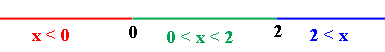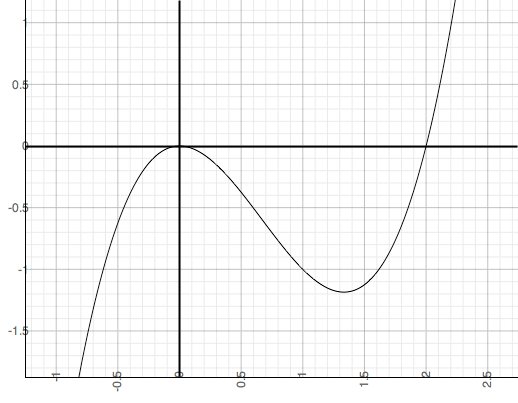SEARCH HOMEMath Central Quandaries & QueriesQuestion from Katie, a student: How do you solve for x when (x^4)-x is greater than or equal to 0? ThanksHi Katie,

I am going to answer your question but for the expression x3 - 2x2.

I think about this question in terms of the graph of the expression y = x3 - 2x2. I want to know when the graph lies above the x-axis (that is when x3 - 2x2 > 0), when it is below the x-axis (that is when x3 - 2x2 < 0) and when it intersects the x-axis (that is when x3 - 2x2 = 0).

To determine when x3 - 2x2 = 0 I factor x3 - 2x2 to obtain x3 - 2x2 = x2(x - 2) and hence x3 - 2x2 = x2(x - 2) = 0 when x = 0 and when x = 2. These two x-values divide the x-axis into three piecesI now choose an x-value in the leftmost piece, x < 0. I want an x-value that is easy to calculate with so I choose x = -1. At x = -1, y = x3 - 2x2 = (-1)3 - 2(-1)2 = -1 -2 = -3. Thus the graph of y = x3 - 2x2 is below the x-axis at x = -1. The important point here is that the graph is thus below the x-axis for all x satisfying x < 0 since to go above the x-axis the graph would need to cross the x-axis and it doesn't touch the x-axis until x = 0.

Now choose a point in the next piece of the axis, 0 < x < 2. Again I want an easy point so take x = 1. At x = 1, y = x3 - 2x2 = 13 - 2(1)2 = 1 -2 = -1. Thus the graph of y = x3 - 2x2 is below the x-axis at x = 1 and thus below the x-axis for all x in 0 < x < 2 since it doesn't touch the x-axis again until x = 2.

Finally choos a point in the remaining piece of the x-axis, 2 < x. This time I take x = 3 and at x = 3, y = x3 - 2x2 = 33 - 2(3)2 = 27 - 18 = 9 and the graph is above the x-axis at x = 3 and therefore above the x-axis fo all x satisfying 2 < x.

Thus my answer is x3 - 2x2 ≥ 0 for 2 ≤ x and for x = 0.

Just as a check here is the graph of y = x3 - 2x2.PennyMath Central is supported by the University of Regina and The Pacific Institute for the Mathematical Sciences.